• mnist手写数字识别模型
2022-07-19 20:52:38

1、训练模型

import tensorflow as tf
from tensorflow.keras import models, layers, optimizers
import matplotlib.pyplot as plt

mnist = tf.keras.datasets.mnist

(x_train, y_train), (x_test, y_test) = mnist.load_data()
x_train, x_test = x_train / 255.0, x_test / 255.0  #归一化
#print(x_train.shape,x_test.shape,y_train.shape,y_test.shape)
y=tf.one_hot(y_train,depth=10)  #depth ont-hot 编码的长度
#print(y)

#######将图片和对应的号码显示出来
# def show_single_image(img_arr, label):
#     plt.imshow(img_arr, cmap='binary')
#     plt.title('%i' % label)
#     plt.show()
#
#
# image_id = 8
# show_single_image(x_train[image_id], y_train[image_id])
model = models.Sequential([layers.Conv2D(filters=6, kernel_size=3, strides=1, input_shape=(28, 28, 1)),
layers.MaxPooling2D(pool_size=2, strides=2),
layers.ReLU(),
layers.Conv2D(filters=16, kernel_size=3, strides=1),
layers.MaxPooling2D(pool_size=2, strides=2),
layers.ReLU(),
layers.Flatten(),
layers.Dense(120, activation='relu'),
layers.Dropout(0.5),
layers.Dense(84, activation='relu'),
layers.Dense(10, activation='softmax')
])
loss=tf.keras.losses.SparseCategoricalCrossentropy(from_logits=False),
metrics=['sparse_categorical_accuracy'])
model.fit(x_train, y_train, epochs=5,validation_data=(x_test, y_test),validation_freq=1)
model.summary()
test_loss, test_acc=model.evaluate(x_test,  y_test)

#print(test_loss, test_acc)
model.save('my_mnist.h5')


2、测试自己手写数字

import tensorflow as tf
import numpy as np

def preprocess_image(image):
image = tf.image.decode_jpeg(image, channels=1)
image = tf.image.resize(image, [28, 28])
image /= 255.0  # normalize to [0,1] range
image = tf.reshape(image, [28, 28, 1])
return image

return preprocess_image(image)

filepath = 'hh.jpg'
test_my_img = (np.expand_dims(test_my_img, 0))
my_result = model.predict(test_my_img)
print('自己的图片预测值 =', np.argmax(my_result))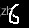输出：自己的图片预测值 = 6python tensorflow
更多相关内容
• 主要为大家详细介绍了Tensorflow训练MNIST手写数字识别模型，文中示例代码介绍的非常详细，具有一定的参考价值，感兴趣的小伙伴们可以参考一下
• 使用MNIST数据集训练手写数字识别模型——**附完整代码**和**训练好的模型文件**——直接用. 具体内容可看我的这篇文章：https://blog.csdn.net/weixin_45954454/article/details/114455209
• 主要为大家详细介绍了基于TensorFlow的CNN实现Mnist手写数字识别，文中示例代码介绍的非常详细，具有一定的参考价值，感兴趣的小伙伴们可以参考一下
• 基于华为自研MindSpore深度学习框架构建网络模型，实现MNIST手写识别实验。 包含自己手写体测试； 包含程序流程图 ； 包含可运行源码、运行结果演示视频，本地MindSpore详细配置教程（私信可远程配置）； 包含联系...
• MNIST手写数字识别问题的多层神经网络模型实践 按课程案例，动手完成编码实践。 自行设计一种神经网络模型，并尝试采用不同超参数，让模型的准确率达到97.5%。 提交要求： 1、你认为最优的一次带运行结果的源代码...
• 手写数字识别原理（不强相关）、具体代码实现（算上import和空行一共50行代码不到）、训练出来的模型怎么使用

本文大概分为以下几个部分：手写数字识别原理（不强相关）、具体代码实现（算上import和空行一共50行代码不到）、训练出来的模型怎么使用。

目录

一、手写数字识别原理

二、具体代码实现

三、训练出来的模型怎么使用

## 一、手写数字识别原理

数字手写体识别由于其有限的类别（0~9共10个数字）成为了相对简单 的手写识别任务。DBRHD和MNIST是常用的两个数字手写识别数据集，我们这次使用的就是MNIST。

怎样识别数字呢？这是一个矩阵，每一个点的数值根据我们之前所说的0-255呈现出黑色到白不同的颜色，大家可以看出来这是一个 7 一个 2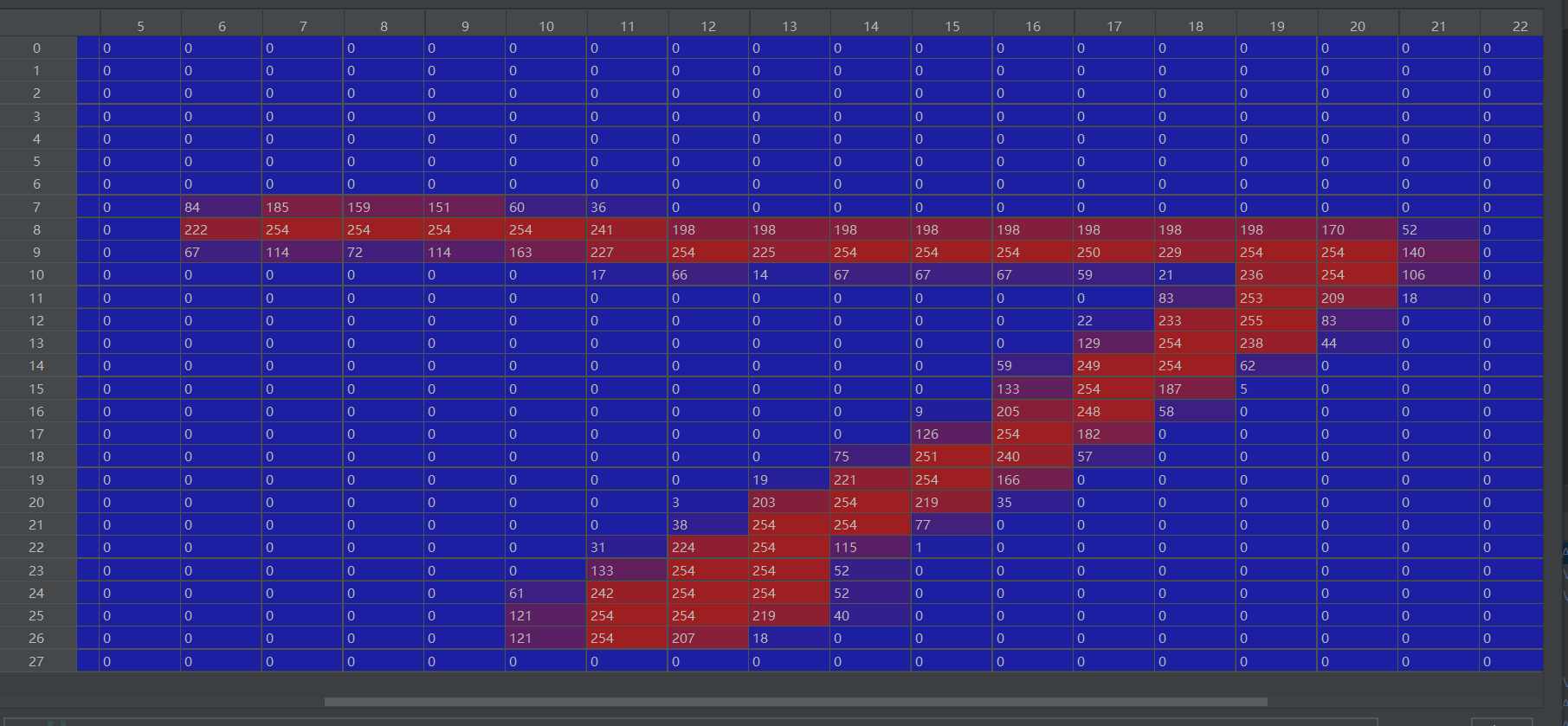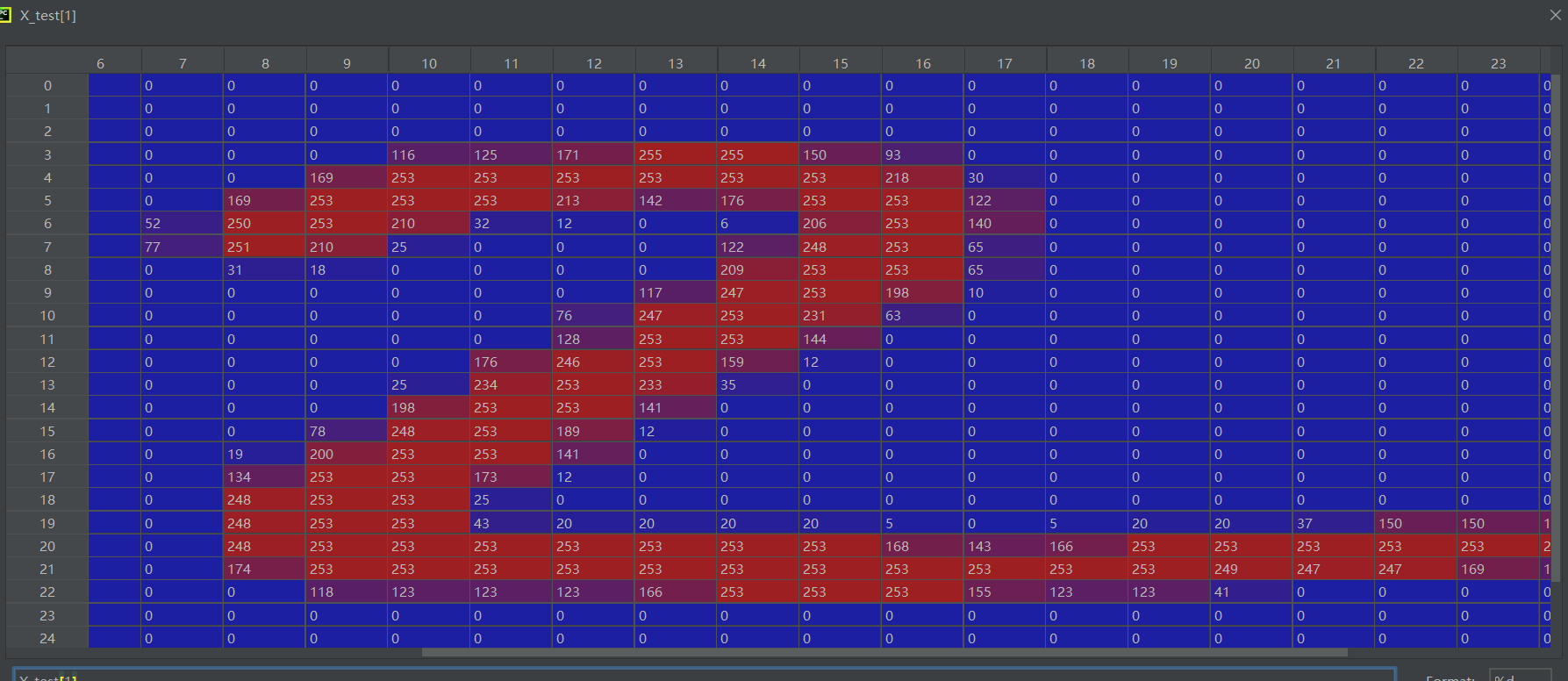你可以通过这个矩阵想象出黑白色上他究竟是什么样子吗（上图中每一个格子都是长方形，所以数字看着显宽，而实际中矩阵的长款都是相等的，所以上图中我们看数字，尽量忽略掉颜色）

它们真实的样子是这样的：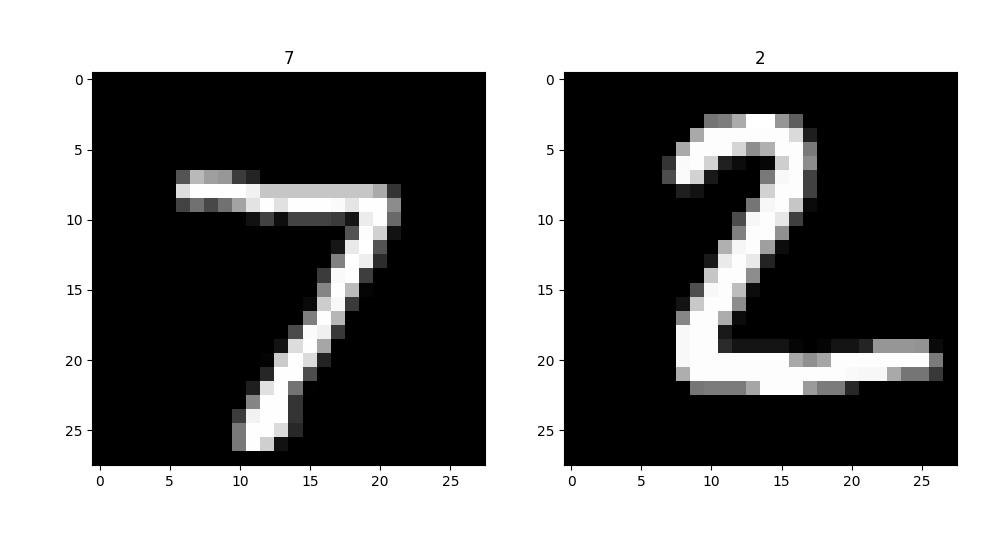我们可以实际对比一下是不是数字越小，颜色越黑，可以注意一下边边角角: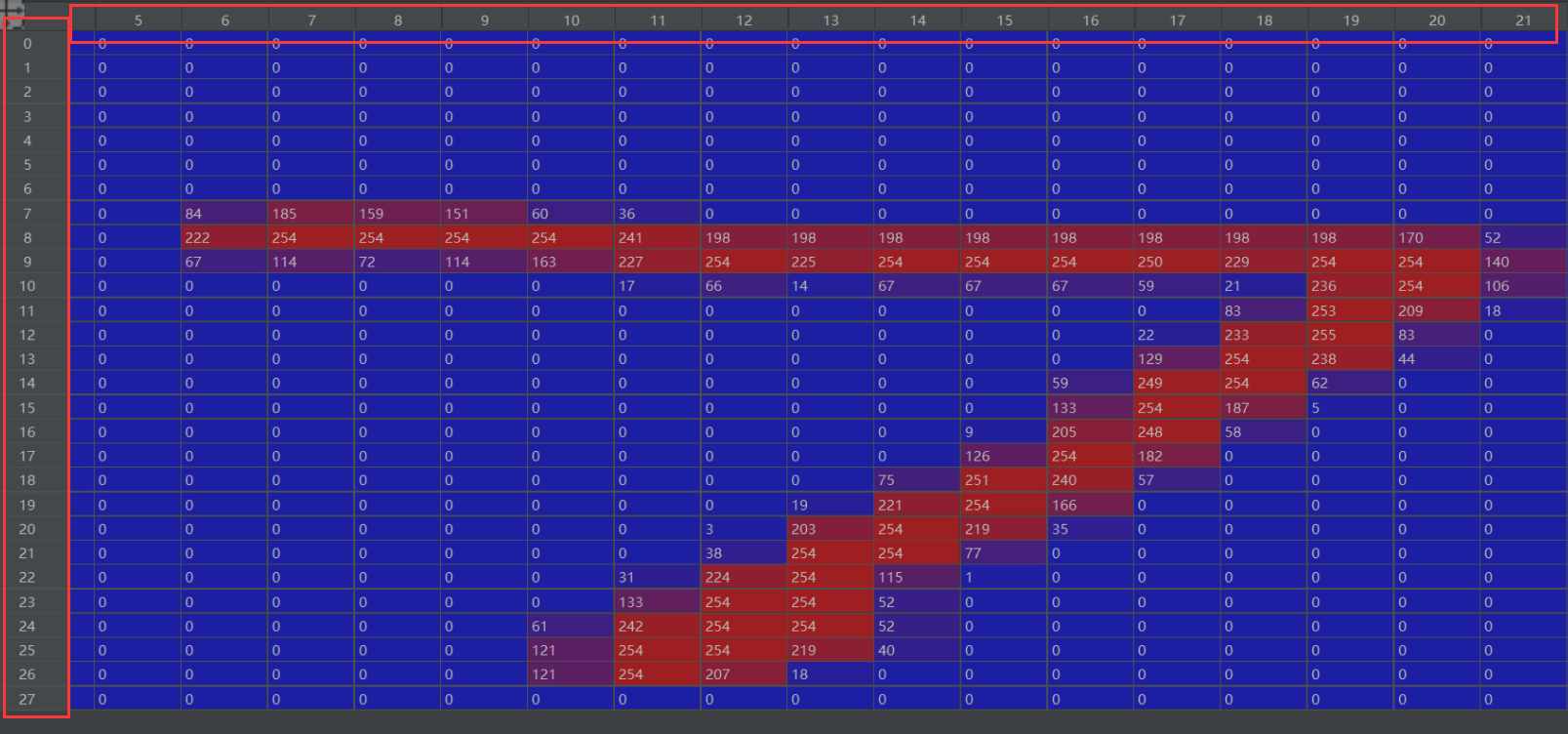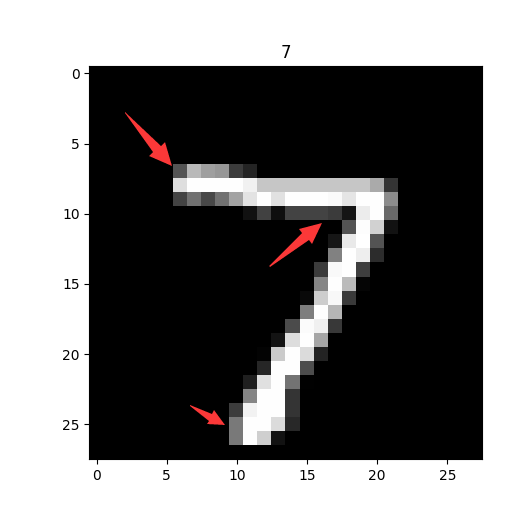而在我们的架构中：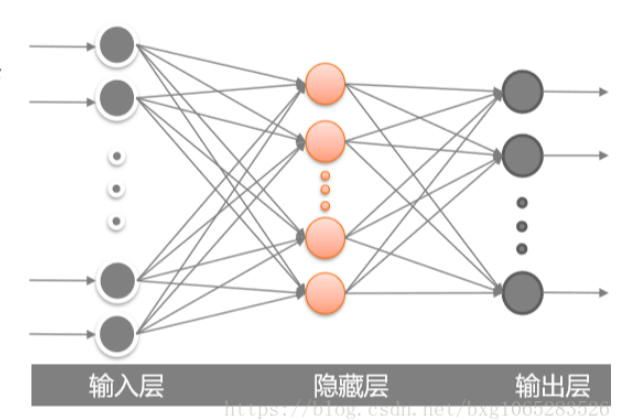机器通过学习0-9的数字走向，最后得出这张图分别是0-9的概率是多大。老规矩，保持兴趣，以后我们再深入理解，现在我们开始动手了！

## 二、具体代码实现

先让我们看一看我们的数字：没有注释的地方都在前两天已经说过哦，不重复解释了

import tensorflow as tf
import numpy as np
import matplotlib.pyplot as plt
from tensorflow.keras import datasets,layers,optimizers,losses

def show_pic(X_train,Y_train):
pic_arr = X_train[:9,:,:]
pic_ind = Y_train[:9]
plt.figure()
for i in range(9):
plt.subplot(3, 3, i+1)
plt.imshow(pic_arr[i,:,:],cmap='gray')
plt.title(str(pic_ind[i]))
plt.show()

//获取Keras自带的mnist数据集分为训练集（(X_train,Y_train)）和验证集（(X_test,Y_test)）

show_pic(X_train,Y_train)
show_pic(X_test,Y_test)

其中X对应的是矩阵，也就是图像；而Y对应的则是图像的数字。比如一张图像 X 是数字9的矩阵，则对应的 Y 就是9这个数字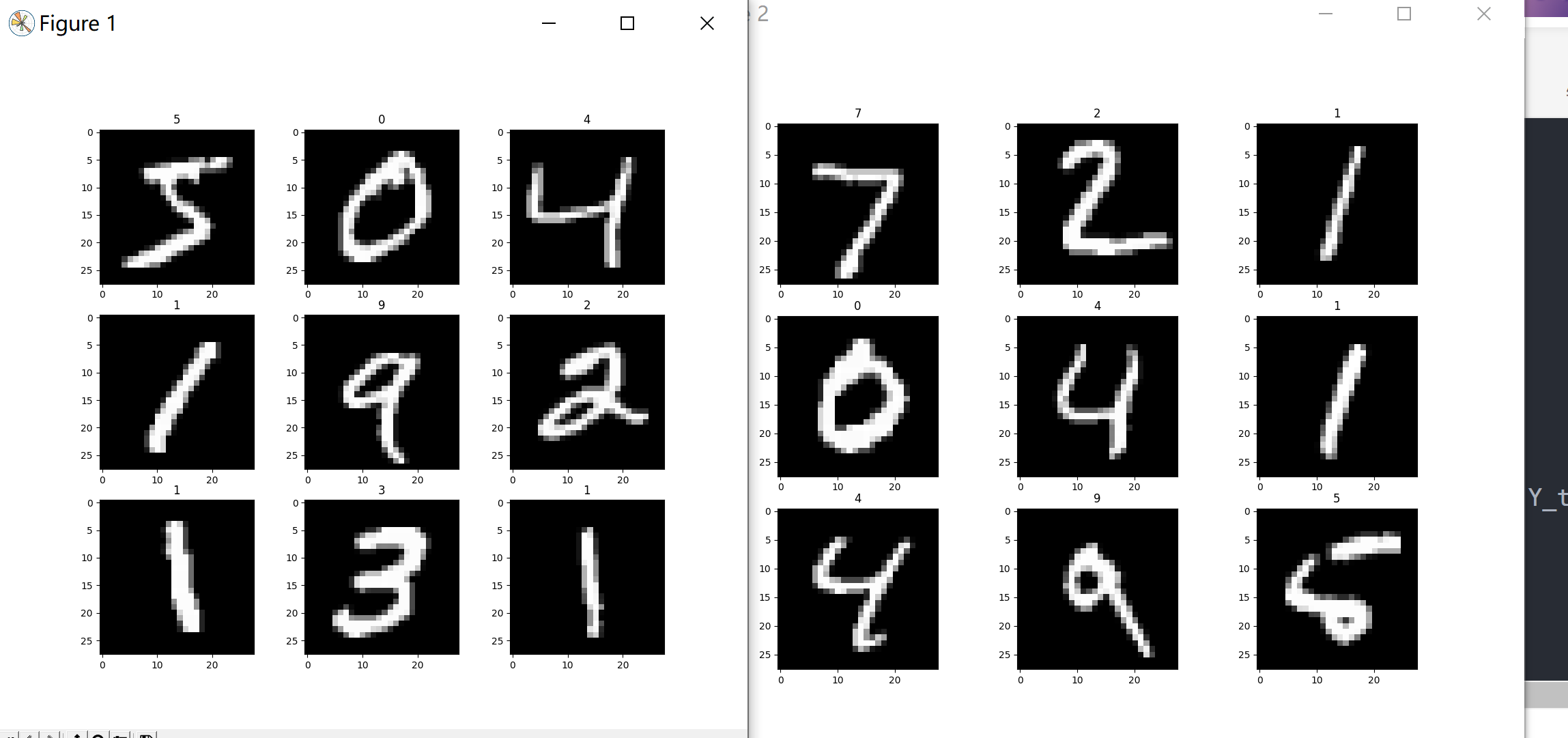看到这些数字了，接下来我们训练模型，在刚才的代码块后面：

X_train = np.reshape(X_train,[60000,28*28])
X_test = np.reshape(X_test,[10000,28*28])

y_train = title_Y(Y_train,10)
y_test = title_Y(Y_test,10)

//建立一个模型，里面是三层全连接，格式都是固定的，最后一次的数字由于我有0-9十个选项，所以写10
model = tf.keras.Sequential([
layers.Dense(700, activation='relu'),
layers.Dense(350,activation='relu'),
layers.Dense(10,activation='softmax')
])

//定义损失和优化函数，不懂原理的可以直接这么写，之前也有介绍
opt = optimizers.SGD(learning_rate=0.001)
loss = losses.CategoricalCrossentropy()

//创建这个模型
model.build(input_shape=[None,784])
//定义优化，损失，准确率
model.compile(optimizer=opt,loss=loss,metrics=['accuracy'])
//训练次数，执行到这一句，就开始训练了
model.fit(x=X_train,y=y_train,batch_size=100,epochs=25,validation_data=(X_test,y_test))

准确率会逐步提升，当然也有可能下降一段时间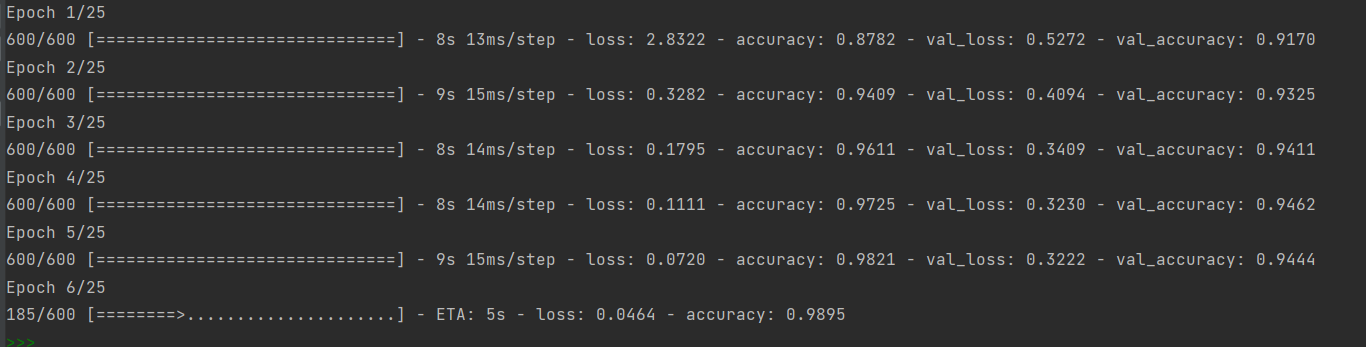## 三、训练出来的模型怎么使用

首先用save()将我们的模型全部保存下来，.h5是专属后缀

model.save('model.h5')

之后我们可以使用这个命令导入我们曾经保存的模型：

model = tf.keras.models.load_model('model.h5')

这样就可以对单独一张图进行预测了，当然，具体是保存整个模型，还是只保存权重，后来发现也是有不一样的，各有优势，更多的模型保存问题和选择会在下一篇详细介绍。

更新：

深度学习TensorFlow---保存和加载 Keras 模型_昊昊该干饭了的博客-CSDN博客目录Keras简介保存整个模型保存模型的权重Keras简介Keras 模型由多个组件组成：架构或配置，它指定模型包含的层及其连接方式。一组权重值（即“模型的状态”）。优化器（通过编译模型来定义）。一组损失和指标（通过编译模型或通过调用add_loss()或add_metric()来定义）。我们可以通过 Keras API 将这些片段一次性保存到磁盘，或仅选择性地保存其中一些片段：将所有内容以 TensorFlow SavedModel 格式（或较早的...
https://blog.csdn.net/qq_52213943/article/details/123842076

写在最后：思考与学习的过程可能会很难，也很慢，你在短时间内也不一定能想清楚，它可能需要花费你1个月、1年，甚至更长的时间...但是，你千万别放弃，它值得你用那么长的时间来思考，因为一旦想清楚，你的人生可能就会从此不同！

你有多长时间只顾低头赶路而忽略了路边的风景呢？

展开全文python 计算机视觉 机器学习 神经网络
• 基于华为自研MindSpore深度学习框架构建网络模型，实现MNIST手写识别实验。 包含可运行源码、运行结果演示视频，本地MindSpore详细配置教程（私信可远程配置） 本例子会使用MindSpore深度学习框架实现一个简单的...
• 在本笔记中，我们将以多层感知机（multilayer perceptron，MLP）为例，介绍多层神经网络的相关概念，并将其运用到最基础的MNIST数据集分类任务中，同时展示相关代码。本笔记主要从下面四个方面展开： 文章目录1 ...
• 利用keras的深度学习框架，分类mnist手写数字识别数据集 二. 实验内容 1.获得mnist数据，并将mnist数据处理成合适的格式; 2.构建神经网络模型: 3.配置训练参数； 4.训练网络； 5.测试训练好的网络模型 三.概要设计 1...

## 卷积神经网络实现

这个代码的话是跟着B站博主敲得 里面也解析了代码 想深入了解的可以看看
链接地址

#### 一. 实验目的

利用keras的深度学习框架，分类mnist手写数字识别数据集

#### 二. 实验内容

1.获得mnist数据，并将mnist数据处理成合适的格式;
2.构建神经网络模型:
3.配置训练参数；
4.训练网络；
5.测试训练好的网络模型

#### 三.概要设计

1.实验原理
①获得mnist数据，并将数据处理成合适的格式:
首先查看数据集是怎样的，让图片由二维铺开成一维，相当于将图片从二维矩阵（28*28）到784像素值的一个向量，同时将数据格式转换为浮点型;
②按照自己的设计搭建神经网络：③设定合适的参数训练神经网络:
a.编译：确定优化器和损失函数;
b.训练网络：确定训练的数据、训练的轮数和每次训练的样本数等；
④在测试集上评价训练效果：
a.利用损失函数变化和训练集的准确率评估它在数据集上的表现：
损失函数逐渐下降，训练集的准确率逐渐提升;
b.用训练好的模型进行预测，并在测试集上进行评价。
2．网络作用
神经网络是机器学习诸多算法中的一种，它既可以用来做有监督的任务，如分类、视觉识别等，也可以用作无监督的任务。同时它能够处理复杂的非线性问题，它的基本结构是神经元。，如下图所示：其中，x1、x2、x3代表输入，中间部分为神经元，而最后的hw,b(x)是神经元的输出。整个过程可以理解为输入——>处理——>输出。
由多个神经元组成的就是神经网络如图所示：
这是一个4层结构的神经网络，layer1为输入层，layer4为输出层，layer2,layer3为隐藏层，即神经网络的结构由输入层，隐藏层，输出层构成。其中除了输入层以外，每一层的输入都是上一层的输出。
而现在所用的卷积神经网络是一种深度学习模型或类似于人工神经网络的多层感知器，常用来分析视觉图像。卷积神经网络能够接受多个特征图作为输入，而不是向量。
3．各个网络层的功能描述
第一层——输入层：
输入层每个神经单元直接对应原始数据，然后向隐藏层提供信息，图片的每一个像素都需要输入层神经元与之对应，对原始图像数据进行预处理，而我们的每个图片大小包含784像素，输入层需要784个神经元；
第二层——隐藏层（中间层）：
隐藏层每个神经单元对不同的输入层神经单元有不同的权重，从而偏向于对某种识别模式兴奋，隐藏层为15个神经元；
第三层——输出层：
多个隐藏层的神经单元兴奋后，输出层的神经单元根据不同隐藏层的兴奋加上权重后，给到不同的兴奋度，这个兴奋度就是模型最终识别的结果，它的神经元个数是确定的，一共为10类，10个神经元

#### 四．详细设计

1．网络结构图
（用 print(network.summary()) 打印）2．各个参数设计

①修改训练集及测试集的图片(28*28像素)从二维矩阵到一维向量:
train_images = train_images.reshape((60000, 28, 28, 1)).astype(‘float’) / 255
test_images = test_images.reshape((10000, 28, 28, 1)).astype(‘float’) / 255
将数据格式转换为浮点型
②训练网络参数:
network.fit(train_images, train_labels, epochs=10, batch_size=128, verbose=2)
epochs:训练多少个回合，
batch_size:每次训练给多大的数据
verbose = 2 为每个epoch输出一行记录
③变量类型及大小:#### 五．测试数据及运行结果

1．正常测试数据和运行结果
①取前五个图片进行预测(结果正常):②取前10个图片进行预测(结果正常)：3．实验结果分析
通过多轮测试集的测试,发现训练效果基本符合,并且打印出训练集和测试集的准确率发现拟合基本相符,准确率如图所示:#### 六．调试情况，设计技巧及体会

1．改进方案
①实验一开始训练集和测试集的准确率低:原来隐藏层个数为15,现在增加到84;
②训练集比测试集拟合的更好:使用正则化 dropout解决
2．体会
一开始根据老师发的教程进行安装，发现运行起来导入库的速度较慢，于是在网上搜索发现Anaconda是一个集成库，于是便开始了安装它，过程比较艰辛，索性安装成功了，最后跑代码的结果很快。
在运行代码的过程中，发现版本更新运行后代码也发生了相应的变化，对此进行了改进。本次应用的是卷积神经网络，它常用来分析视觉图像，十分符合我们这次的实验要求。每次不同的课题，我们对网络模型的选择也是非常重要的。
这个实验我们在课上做了两三次，每次运行代码都会有新的改进收获匪浅。通过CSDN博客，我学习到了tensorflow框架的基本使用方法，通过python和tensorflow进行了神经网络的构造和实现，并通过神经网络实现了手写数字识别的功能。
通过这次实验，我觉得机器学习是个特别有意思的学科，可以进行爬虫和数据预测等，通过自己的努力看到实验的准确率稳步上升，成就感满满。在以后的学习生活中，我会以更大的兴趣投入其中，并深入学习神经网络的内容。fighting!!!

代码实现：

from tensorflow.keras.utils import to_categorical
from tensorflow.keras import models, layers
from tensorflow.keras.optimizers import RMSprop
from tensorflow.keras.datasets import mnist
# 加载数据集
(train_images, train_labels), (test_images, test_labels) = mnist.load_data()

# 搭建LeNet网络 神经网络的模型
def LeNet():
network = models.Sequential()
network.add(layers.Conv2D(filters=6, kernel_size=(3, 3), activation='relu', input_shape=(28, 28, 1)))
network.add(layers.Conv2D(filters=16, kernel_size=(3, 3), activation='relu'))
network.add(layers.Conv2D(filters=120, kernel_size=(3, 3), activation='relu'))
return network
network = LeNet()
network.compile(optimizer=RMSprop(lr=0.001), loss='categorical_crossentropy', metrics=['accuracy'])

#将图片由二维铺开成一维（相当于将图片从二维矩阵到一维向量）
train_images = train_images.reshape((60000, 28, 28, 1)).astype('float') / 255
test_images = test_images.reshape((10000, 28, 28, 1)).astype('float') / 255
train_labels = to_categorical(train_labels)
test_labels = to_categorical(test_labels)
print(test_labels)
# 训练网络，用fit函数, epochs表示训练多少个回合， batch_size表示每次训练给多大的数据
network.fit(train_images, train_labels, epochs=10, batch_size=1280, verbose=2)

#print(network.summary())
#测试集测试模型性能 取前五张图片
y_pre = network.predict(test_images[:10])
print(y_pre,test_labels[:10])
test_loss, test_accuracy = network.evaluate(test_images, test_labels)
print("test_loss:", test_loss, "    test_accuracy:", test_accuracy)


展开全文keras 神经网络
• 手写数字识别的Tensorflow完整代码，### 1. MNIST机器学习入门 **1.1.1 简介** 下载MNIST数据集，并打印一些基本信息：  python download.py  **1.1.2 实验：将MNIST数据集保存为图片**  ...人工智能
• MNIST数据集是机器学习领域中非常经典的一个数据集，由60000个训练样本和10000个测试样本组成，每个样本都是一张28 * 28像素的灰度手写数字图片，且内置于keras。本文采用Tensorflow下Keras（Keras中文文档）神经...

# 数据集介绍

MNIST数据集是机器学习领域中非常经典的一个数据集，由60000个训练样本和10000个测试样本组成，每个样本都是一张28 * 28像素的灰度手写数字图片，且内置于keras。本文采用Tensorflow下Keras（Keras中文文档）神经网络API进行网络搭建。

开始之前，先回忆下机器学习的通用工作流程（ ✅表示本文用到，❌表示本文没有用到 )

1. 定义问题，收集数据集（✅）
2. 选择衡量成功的指标（✅）
3. 确定评估的方法（✅）
4. 准备数据（✅）
5. 开发比基准更好的模型（❌）
6. 扩大模型规模（❌）
7. 模型正则化与调节参数（❌）

关于最后一层激活函数与损失函数的选择

问题类型最后一层激活函数损失函数
二分类sigmoidbinary_crossentropy
多分类、单标签softmaxcategorical_crosentropy
多分类、多标签sigmoidbinary_crossentropy
回归到任意值mse
回归到0～1之间sigmoidmse或binary_crossentropy

下面开始正文～

# 1.数据预处理

我们来看看它的源码声明：

def load_data(path='mnist.npz'):
"""Loads the [MNIST dataset](http://yann.lecun.com/exdb/mnist/).

This is a dataset of 60,000 28x28 grayscale images of the 10 digits,
along with a test set of 10,000 images.
More info can be found at the
[MNIST homepage](http://yann.lecun.com/exdb/mnist/).

Arguments:
path: path where to cache the dataset locally
(relative to ~/.keras/datasets).

Returns:
Tuple of Numpy arrays: (x_train, y_train), (x_test, y_test).
**x_train, x_test**: uint8 arrays of grayscale image data with shapes
(num_samples, 28, 28).

**y_train, y_test**: uint8 arrays of digit labels (integers in range 0-9)
with shapes (num_samples,).
"""



可以看到，里面包含了数据集的下载链接，以及数据集规模、尺寸以及数据类型的声明，且函数返回的是四个numpy array组成的两个元组。

导入数据集并reshape至想要形状，再标准化处理。
其中内置于keras的to_categorical()就是one-hot编码——将每个标签表示为全零向量，只有标签索引对应的元素为1.

eg: col=10

[0,1,9]-------->[ [1,0,0,0,0,0,0,0,0,0],
[0,1,0,0,0,0,0,0,0,0],
[0,0,0,0,0,0,0,0,0,1] ]


我们可以手动实现它：

def one_hot(sequences,col):
resuts=np.zeros((len(sequences),col))
# for i,sequence in enumerate(sequences):
#         resuts[i,sequence]=1
for i in range(len(sequences)):
for j in range(len(sequences[i])):
resuts[i,sequences[i][j]]=1
return resuts


下面是预处理过程

def data_preprocess():
(train_images, train_labels), (test_images, test_labels) = mnist.load_data()
train_images = train_images.reshape((60000, 28, 28, 1))
train_images = train_images.astype('float32') / 255
#print(train_images)
test_images = test_images.reshape((10000, 28, 28, 1))
test_images = test_images.astype('float32') / 255

train_labels = to_categorical(train_labels)
test_labels = to_categorical(test_labels)
return train_images,train_labels,test_images,test_labels


# 2.网络搭建

这里我们搭建的是卷积神经网络，就是包含一些卷积、池化、全连接的简单线性堆积。我们知道多个线性层堆叠实现的仍然是线性运算，添加层数并不会扩展假设空间（从输入数据到输出数据的所有可能的线性变换集合），因此需要添加非线性或激活函数。relu是最常用的激活函数，也可以用prelu、elu

def build_module():
model = models.Sequential()
#第一层卷积层，首层需要指出input_shape形状
model.add(layers.Conv2D(32, (3,3), activation='relu', input_shape=(28,28,1)))
#第二层最大池化层
#第三层卷积层
#第四层最大池化层
#第五层卷积层
#第六层Flatten层，将3D张量平铺为向量
#第七层全连接层
#第八层softmax层，进行分类
return model


使用model.summary()查看搭建的网路结构：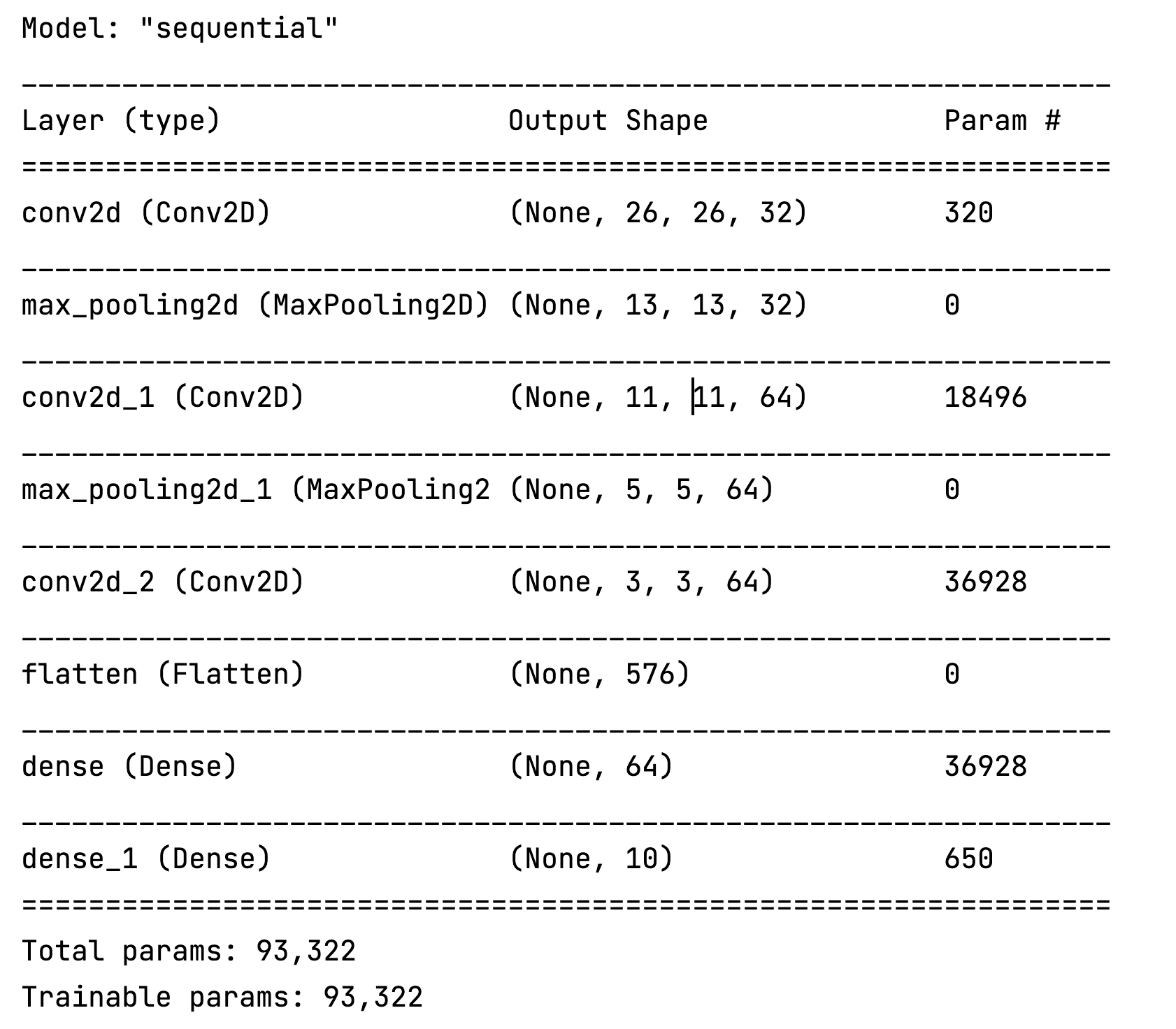# 3.网络配置

网络搭建好之后还需要关键的一步设置配置。比如：优化器——网络梯度下降进行参数更新的具体方法、损失函数——衡量生成值与目标值之间的距离、评估指标等。配置这些可以通过 model.compile() 参数传递做到。
我们来看看model.compile()的源码分析下：

  def compile(self,
optimizer='rmsprop',
loss=None,
metrics=None,
loss_weights=None,
weighted_metrics=None,
run_eagerly=None,
steps_per_execution=None,
**kwargs):
"""Configures the model for training.

参数含义
loss损失函数：mean_squared_error、mean_absolute_error、mean_absolute_percentage_error、mean_squared_logarithmic_error、squared_hinge、hinge、categorical_hinge、logcosh
metrics评估模型在训练和测试时的性能指标。典型用法：metrics=[‘accuracy’]

## 关于优化器

优化器：字符串（优化器名称）或优化器实例。
字符串格式：比如使用优化器的默认参数
实例优化器进行参数传入：

keras.optimizers.RMSprop(lr=0.001, rho=0.9, epsilon=None, decay=0.0)
model.compile(optimizer='rmsprop'，loss='mean_squared_error')


建议使用优化器的默认参数 （除了学习率 lr，它可以被自由调节）
参数：

lr: float >= 0. 学习率。
rho: float >= 0. RMSProp梯度平方的移动均值的衰减率.
epsilon: float >= 0. 模糊因子. 若为 None, 默认为 K.epsilon()。
decay: float >= 0. 每次参数更新后学习率衰减值。


这么多优化器、该如何选择呢？各种优化器Optimizer的总结与比较

## 关于损失函数

取决于具体任务，一般来说损失函数要能够很好的刻画任务。比如

1. 回归问题
希望神经网络输出的值与ground-truth的距离更近，选取能刻画距离的loss应该会更合适，比如L1 Loss、MSE Loss等
2. 分类问题
希望神经网络输出的类别与ground-truth的类别一致，选取能刻画类别分布的loss应该会更合适，比如cross_entropy
具体常见选择可查看文章开始处关于损失函数的选择

## 关于指标

常规使用查看上述列表即可。下面说说自定义评价函数：它应该在编译的时候（compile）传递进去。该函数需要以 (y_true, y_pred) 作为输入参数，并返回一个张量作为输出结果。

import keras.backend as K
def mean_pred(y_true, y_pred):
return K.mean(y_pred)

model.compile(optimizer='rmsprop',
loss='binary_crossentropy',
metrics=['accuracy', mean_pred])


# 4.网络训练与测试

1. 训练（拟合）
使用model.fit()，它可以接受的参数列表
def fit(self,
x=None,
y=None,
batch_size=None,
epochs=1,
verbose=1,
callbacks=None,
validation_split=0.,
validation_data=None,
shuffle=True,
class_weight=None,
sample_weight=None,
initial_epoch=0,
steps_per_epoch=None,
validation_steps=None,
validation_batch_size=None,
validation_freq=1,
max_queue_size=10,
workers=1,
use_multiprocessing=False):


这个源码有300多行长，具体的解读放在下次。
我们对训练数据进行划分，以64个样本为小批量进行网络传递，对所有数据迭代5次

model.fit(train_images, train_labels, epochs = 5, batch_size=64)

1. 测试
使用model.evaluates()函数
 test_loss, test_acc = model.evaluate(test_images, test_labels)


关于测试函数的返回声明：

Returns:
Scalar test loss (if the model has a single output and no metrics)
or list of scalars (if the model has multiple outputs
and/or metrics). The attribute model.metrics_names will give you
the display labels for the scalar outputs.


# 5.绘制loss和accuracy随着epochs的变化图

model.fit()返回一个History对象，它包含一个history成员，记录了训练过程的所有数据。
我们采用matplotlib.pyplot进行绘图，具体见后面完整代码。

Returns:
A History object. Its History.history attribute is
a record of training loss values and metrics values
at successive epochs, as well as validation loss values
and validation metrics values (if applicable).


点我-》Matplotlib菜鸟教程

def draw_loss(history):
loss=history.history['loss']
epochs=range(1,len(loss)+1)
plt.subplot(1,2,1)#第一张图
plt.plot(epochs,loss,'bo',label='Training loss')
plt.title("Training loss")
plt.xlabel('Epochs')
plt.ylabel('Loss')
plt.legend()

plt.subplot(1,2,2)#第二张图
accuracy=history.history['accuracy']
plt.plot(epochs,accuracy,'bo',label='Training accuracy')
plt.title("Training accuracy")
plt.xlabel('Epochs')
plt.ylabel('Accuracy')
plt.suptitle("Train data")
plt.legend()
plt.show()


# 6.完整代码

from tensorflow.keras.datasets import mnist
from tensorflow.keras import models
from tensorflow.keras import layers
from tensorflow.keras.utils import to_categorical
import matplotlib.pyplot as plt
import numpy as np
def data_preprocess():
(train_images, train_labels), (test_images, test_labels) = mnist.load_data()
train_images = train_images.reshape((60000, 28, 28, 1))
train_images = train_images.astype('float32') / 255
#print(train_images)
test_images = test_images.reshape((10000, 28, 28, 1))
test_images = test_images.astype('float32') / 255

train_labels = to_categorical(train_labels)
test_labels = to_categorical(test_labels)
return train_images,train_labels,test_images,test_labels

#搭建网络
def build_module():
model = models.Sequential()
#第一层卷积层
model.add(layers.Conv2D(32, (3,3), activation='relu', input_shape=(28,28,1)))
#第二层最大池化层
#第三层卷积层
#第四层最大池化层
#第五层卷积层
#第六层Flatten层，将3D张量平铺为向量
#第七层全连接层
#第八层softmax层，进行分类
return model
def draw_loss(history):
loss=history.history['loss']
epochs=range(1,len(loss)+1)
plt.subplot(1,2,1)#第一张图
plt.plot(epochs,loss,'bo',label='Training loss')
plt.title("Training loss")
plt.xlabel('Epochs')
plt.ylabel('Loss')
plt.legend()

plt.subplot(1,2,2)#第二张图
accuracy=history.history['accuracy']
plt.plot(epochs,accuracy,'bo',label='Training accuracy')
plt.title("Training accuracy")
plt.xlabel('Epochs')
plt.ylabel('Accuracy')
plt.suptitle("Train data")
plt.legend()
plt.show()
if __name__=='__main__':
train_images,train_labels,test_images,test_labels=data_preprocess()
model=build_module()
print(model.summary())
model.compile(optimizer='rmsprop', loss = 'categorical_crossentropy', metrics=['accuracy'])
history=model.fit(train_images, train_labels, epochs = 5, batch_size=64)
draw_loss(history)
test_loss, test_acc = model.evaluate(test_images, test_labels)
print('test_loss=',test_loss,'  test_acc = ', test_acc)

`

迭代训练过程中loss和accuracy的变化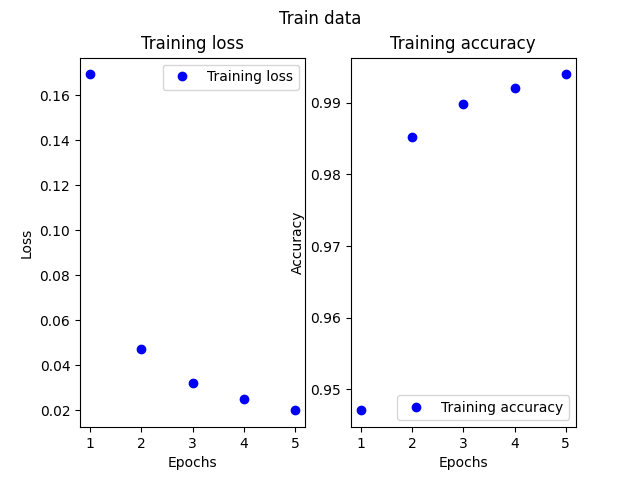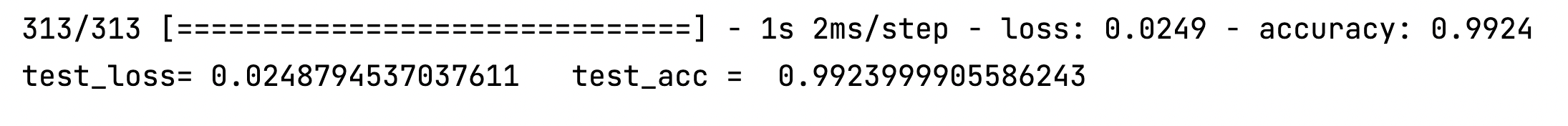由于数据集比较简单，随便的神经网络设计在测试集的准确率可达到99.2%
END！

展开全文机器学习 python
• MNIST手写数字识别我将在PyTorch中构建一个简单的神经网络，并训练它使用MNIST数据集来识别手写数字。 在MNIST数据集上训练分类器可以被视为图像识别的世界。 创建神经网络的步骤如下： 搭建环境准备数据集建立网络...
• 1、Mnist_cnn.py 该脚本文件 用TensorFlow框架 实现CNN卷积神经网络处理Mnist手写数字识别数据集，准确率达到99.21%； 2、Mnist_cnn_tensorboard.py 该脚本文件在Mnist_cnn.py的基础上实现可视化。
• keras实现的mnist手写数字识别模型已经训练好，也可以重新进行训练，带了自己手写的28x28的测试图片，有需要的可以下载
• 第一层需要通过参数传递告知模型数据规格，后边的层不需要，因为可以自动的根据第一层的输出进行推导。 通过input_shape参数： 也可以通过input_dim参数设定，和上边的含义类似： 注意：input_shape=(28✖28,)代表的...tensorflow python
• 手写数字识别（从ipynb转化成py，直接就可运行）： #!/usr/bin/env python # coding: utf-8 # ## 一、加载数据 # x_train表示训练数据集，y_train表示训练数据集对应的结果； # # x_test表示测试数据集，y_test...tensorflow python
• 在完成了MNIST手写数字模型的训练之后，我们就可以使用训练好的模型进行预测手写数字了。这里还是使用MNIST数据集中所提供的测试数据。 可以仅仅对测试集的数据进行预测，并直接打印出来结果即可。但是为了和原图像...python 机器学习 人工智能
•tensorflow
• hello大家好！我又来搬文章了！我就不信还有比这更详细的？...在本文中，我们将在PyTorch中构建一个简单的卷积神经网络，并使用MNIST数据集训练它识别手写数字。在MNIST数据集上训练分类器可以看作是......神经网络 python 图像识别 人工智能
• 主要介绍了Python利用逻辑回归模型解决MNIST手写数字识别问题,结合实例形式详细分析了Python MNIST手写识别问题原理及逻辑回归模型解决MNIST手写识别问题相关操作技巧,需要的朋友可以参考下
• MNIST数据集是由0〜9手写数字图片和数字标签所组成的，由60000个训练样本和10000个测试样本组成，每个样本都是一张28 * 28像素的灰度手写数字图片。MNIST 数据集来自美国国家标准与技术研究所，整个训练集由250个...
•pytorch python
• (3)单次优化：optimizer.step #梯度置0:optimizer.zero_grad() #反向传播:loss.backward() #单次优化：optimizer.step 二、训练 1.MNIST手写数字识别训练流程： 2.交叉熵损失: ...pycharm 计算机视觉 pytorch...